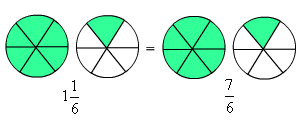# Improper Fractions

An improper fraction is a fraction in which the numerator (top number) is greater than or equal to the denominator (bottom number).  Fractions such as $\frac{6}{5}$ or $\frac{11}{4}$ are “improper”.

Are improper fractions incorrect?  No, they are really just another way of writing a mixed numberFor mathematics, improper fractions are actually easier to use than mixed fractions.  But, for everyday use, people understand mixed numbers better.  So, it is important that you know how to convert from one form to the other.

Example 1:

Write the mixed number $3\frac{1}{4}$ as an improper fraction.

First, write the mixed number as a sum of a whole number and a proper fraction. Then write the two parts with common denominator , and add.

$3+\frac{1}{4}=\frac{12}{4}+\frac{1}{4}=\frac{13}{4}$

Example 2:

Write the improper fraction $\frac{27}{5}$ as a mixed number.

Divide the denominator into the numerator, and rewrite the fraction as a sum of the whole part plus the remainder.

$\frac{27}{5}=5+\frac{2}{5}=5\frac{2}{5}$